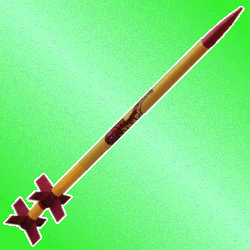Multistage Rocket Equations

Equations for model rocketeers - how to accurately predict speed and altitude for your multistage rocket from weight, diameter, motor thrust and impulse. If you're here I take it you're serious and looking for answers so let's get right to it. I'm assuming you've read and understand the single-stage equations.

## First Stage

The equations to find velocity after first stage boost are the same as single stage boost, that is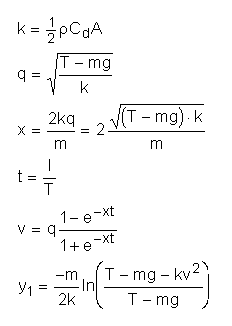notice I call the distance travelled during the first stage boost "y1" instead of "yb". I do that because I'm going to call the second stage distance "y2", the third stage distance "y3" and in general, the boost stages are now called "yn".

## Upper stages

For each stage after the first, you use a generalized version of these equations. First, set

• v0 equal to the end velocity (v) from the last stage
• m equal to the mass of the rocket after the previous stage has been ejected
• I equal to the impulse for the new stage
• T equal to the thrust for the new stage
• k stays the same as it was for the first stage

The following equations don't change but you recalculate with the new values of m, I and T: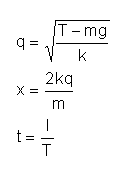Incidentally, the term "q", which is used because it makes the computation easier, also happens to be the terminal velocity for the rocket under thrust. So once you’ve computed q, you know how close your rocket comes to reaching terminal velocity (the point at which the wind resistance equals thrust, that is, you can’t GO faster than this). For single stage rockets this is no big deal because you never come close, but for multistage rockets, you may very nearly reach terminal velocity during boost.

The next term, s, is new for the upper stages: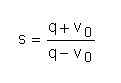Then the next two equations are slightly different for the upper stages. Notice that if v0 = 0, these equations become the same as the first stage, or single stage, equations, exactly as they should: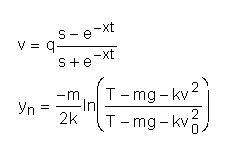The velocity v that you calculate here is the actual velocity of the rocket at the end of the boost phase. The altitude yn is the distance covered only during this boost phase, that is, you have to add up all the y's to get the total altitude the rocket will go.

## Coasting

The coasting equations are the same as before with the single stage rocket, that is,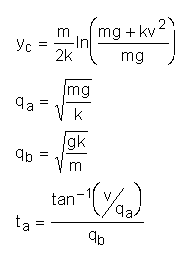Then the total altitude is the sum of each of the altitudes you calculated above, for example, for a three stage rocket the total altitude reached is yt = y1 + y2 + y3 + yc . The coasting time is given by ta, and the total time to apogee is the sum of the burn times plus the coasting time.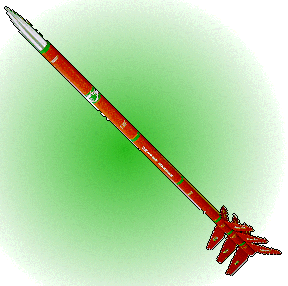Let's Try It Out

We'll go with a Comanche-3, Estes 3-stage rocket, using a D12-0 first stage, C6-0 second stage, and C6-7 third stage. Estes says this rocket will go more than a half-mile, or 2,640 feet. Let's just see about that. The specs on this rocket are:

• mass = 58.9 grams = 0.0589 kg
• diameter = 24.8 mm = 0.0248 m

As I have before, I will use

• rho = 1.2
• Cd = 0.75

A reasonable approximation is to take the weight of the motor to be the weight of the stage. That is, the body tube, tube coupler, and fins on each stage weigh about 3 grams, which can be considered negligible compared to the weight of the booster motor itself. So to compute the weight of the stage I will used the following data, which you can get from an Estes catalog or from here:

• D12-0: initial mass - 40.9 g; mass of propellant - 24.93 g
• C6-0: initial mass - 22.7 g; mass of propellant - 12.48 g
• C6-7: initial mass - 26.9 g; mass of propellant - 12.48 g

As I have done before, I will compute the mass of the whole rocket as the rocket's empty mass, plus all the motors still on board, minus half the propellant weight for the motor that's burning. During the coast phase I will use the weight of the upper stage minus its entire propellant weight (since it's still there and burned out). So the masses for each stage work out to be

• During first stage burn: 0.0589 + 0.0269 + 0.0227 + 0.0409 - 0.02493/2 = 0.136935
• During second stage burn: 0.0589 + 0.0269 + 0.0227 - 0.01248/2 = 0.10226
• During third stage burn: 0.0589 + 0.0269 - 0.01248/2 = 0.07956
• During coast phase: 0.0589 + 0.0269 - 0.01248 = 0.07332

Also - for D engines the rating, taken from Model Rocket Motor Dimension WWW Page, is 0.85, so the actual D engine impulse is 20*0.85 = 17 N-s. As I have done before, I use the rating of 0.9 for the C engines, so they have an impulse of 9 N-s.

Then the calculations go like this:

For the first stage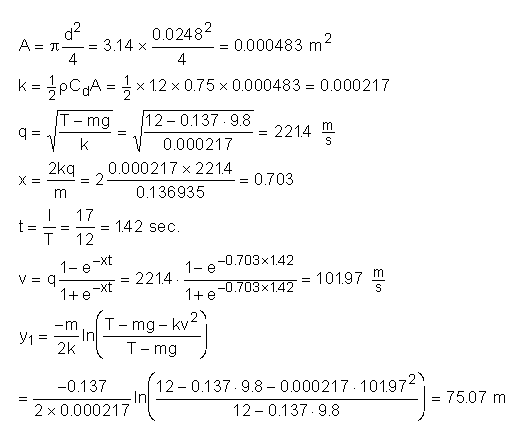Second Stage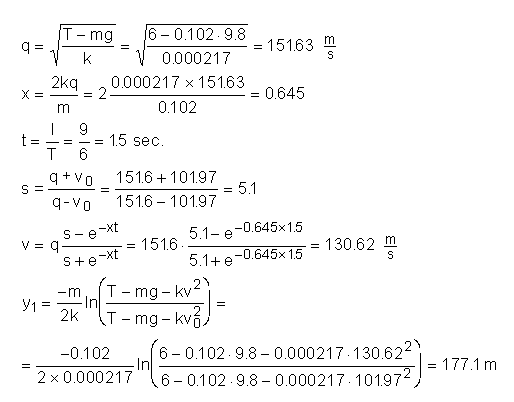Third Stage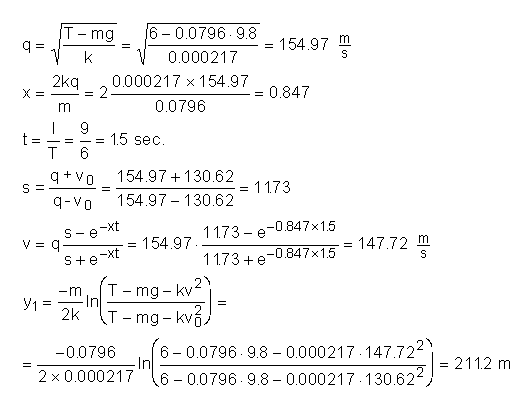Notice that the rocket comes within about 7 m/s of terminal velocity q on the way up.

Coasting Phase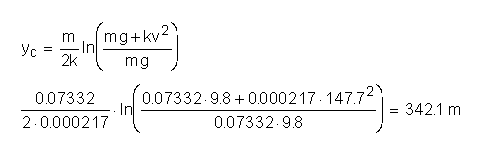So the peak altitude by our reckoning is

yt = y1 + y2 + y3 + yc = 75.1 + 177.1 + 211.2 + 342.1
= 805.5 meters = 2,642 feet

which is indeed greater than 2,640 feet, by two feet. It really does go higher than a half-mile.

Coast Time

How much of a delay should the last stage really have? We assumed a C6-7 engine, with a 7-second delay, as recommended by Estes. Using the equations for coast time we can check that: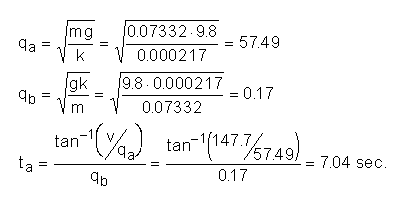So 7 seconds is indeed the right delay to use.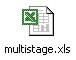Handy Excel Spreadsheet for 3-stage calculation.

## Questions

Your questions and comments regarding this page are welcome. You can e-mail Randy Culp (Tripoli #6926) for inquiries, suggestions, new ideas or just to chat.
Updated 24 August 2008